# Effect of Contour Shape of Nervous System Electromagnetic Stimulation Coils on the Induced Electrical Field Distribution

## Abstract

### Background

Electromagnetic stimulation of the nervous system has the advantage of reduced discomfort in activating nerves. For brain structures stimulation, it has become a clinically accepted modality. Coil designs usually consider factors such as optimization of induced power, focussing, field shape etc. In this study we are attempting to find the effect of the coil contour shape on the electrical field distribution for magnetic stimulation.

### Method and results

We use the maximum of the induced electric field stimulation in the region of interest as the optimization criterion. This choice required the application of the calculus of variation, with the contour perimeter taken as a pre-set condition. Four types of coils are studied and compared: circular, square, triangular and an 'optimally' shaped contour. The latter yields higher values of the induced electrical field in depths up to about 30 mm, but for depths around 100 mm, the circular shape has a slight advantage. The validity of the model results was checked by experimental measurements in a tank with saline solution, where differences of about 12% were found. In view the accuracy limitations of the computational and measurement methods used, such differences are considered acceptable.

### Conclusion

We applied an optimization approach, using the calculus of variation, which allows to obtain a coil contour shape corresponding to a selected criterion. In this case, the optimal contour showed higher intensities for a longer line along the depth-axis. The method allows modifying the induced field structure and focussing the field to a selected zone or line.

## Background

The Electromagnetic stimulation was first applied for excitation of peripheral nerves  and later for transcranial brain excitation to evoke motor responses . The latter has become a routinely accepted diagnostic procedure. Various other applications have been reported . An optimization of the circular coil winding for brain excitation was proposed by Ruohonen et al . Respiration stimulation was attempted in animal and man [5, 6], but did not found practical application. Magnetic pacing of the closed chest dog heart was also undertaken [7, 8], but proved to require extremely high energy. Open chest dog heart stimulation was achieved with lower thresholds . Some theoretical considerations for magnetic field cardiac excitation in man have been considered by .

Electromagnetic stimulation in all of the above-cited studies was generated with circular coils. Combinations of circular coils – butterfly, four-leaf, or slinky type [11, 12], were designed for better focussing and/or increased energy. The newly proposed 'slinky' coils promise better performances in this respect .

Square slinky coils were considered by Lin et al , yielding a better expressed (hence better focussed) but lower field intensity maximum, compared to circular coils.

Having in view that better focussing and improved field distribution would provide for lower energy requirements, we decided to investigate whether different coil shapes would yield some improvement in this sense.

## Method

### 2.1. Theoretical background

A natural criterion for optimal coil design would be the electrical field induced in the region of interest. It is defined by: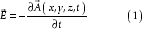whereis the induced field andis the magnetic vector potential obtained from the coil current pulses.

Electromagnetic stimulation processes could be studied using a more general relation: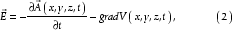where the electric potential component is added. However, it is usually neglected due to its relatively low value and hence weak influence. For a given coil current i(t) and contour shape (S), the magnetic vector-potential is defined by the known relation: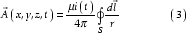For the induced field and a plane contour placed in the plane XOY (Fig. 1),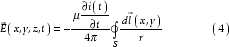The necessary and sufficient conditions for finding the extremum of Eq. (4) should be defined, for a known time-course of the current i(t). Thus, the induced field at point M (Fig. 1) will be at its maximum .

The function y(x) has to be found by solving the relation: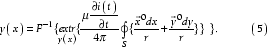There are reasons to assume that a real solution of the above problem exists, and that it respects the condition of sufficiency.

The conditions for existence of extremum of Eq.(4), concerning y(x), can be defined by the function: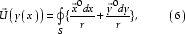which is of simple structure, but has the following peculiarities:

• a non-standard expression where the extremum function y(x) is hidden in the integral boundary;

• a vector function where the application of the classical calculus of variation approach is inapplicable without some necessary transformations.

The conversion of Eq. (6) to a standard form is shown in Appendix A, which contains the succession of transformations leading to the necessary conditions for existence of extremum, respectively, to the Euler equations:This system yields a stable numeric solution as a Cauchy problem, for a Lagrange constant value of λ = 1.5 and boundary conditions regarding the quantities x 1 (t)t = 0 = 0.0025; x 2 (t)t = 0 = 0.1; x'1 (t)t = 0 = 4; x'2 (t)t = 0 = 400. The extremal appears as shown in Fig. 2.

Analyzing the obtained solution, it should be taken into account that the axes scaling in Fig. 2 is influenced by the canceling of the multipliers to the functions of Eqs. (3) and (4).

A solution could be attempted not only as a Cauchy type, but also as a Sturm-Liouville problem, with the corresponding fundamental difficulties.

The extremal y(x) of Fig. 2 is considered in the class of symmetric curves with respect to OX, which is natural for this type of problem. The solution of the Cauchy problem in the class of closed curves creates difficulties with the use of numerical procedures. This is due do reaching, generally, points of unstable solution, where the first derivative is interrupted. Thus, it becomes necessary to look for the extremal in parametric form.

In addition, it can be shown that the necessary conditions considered are also sufficient (see Appendix B for details).

## Results and discussion

The shape of the obtained extremal is approximated by linear segmentation (Fig. 3), respecting the condition for a given constant perimeter.

The field excited by a system of three coils located symmetrically with respect to the ordinate in the XOY and YOZ planes is studied, using the basic relation of Eq. (4) (see Appendix C for details).

It can be seen that for a field excited by a system of coils, the superposition principle is applicable, due to the linear relation between the cause di(t)/dt (applied excitation) and the consequence E(t) (resultant field).

Thus, the results obtained for a given coil shape can be applied for a system of several coils of the same type.

The distribution of the fields E(x) in the XOY plane for y = 0, excited by triads of circular, square, triangular and optimal contour coils, are shown in Fig. 4. The shape approximating an optimal contour was obtained by the variation method. The induced electrical fields E(xy) distributions in the XOY plane for a distance Z = 5 mm from the coordinate system origin are shown for the four types of coils in Figs. 5,6,7,8, respectively.

The excitation is obtained by the discharge current of an RLC circuit, where R = 0.3 Ω, L = 0.01 mH and C = 128 μF. These parameters are chosen in a relatively arbitrary way, and do not influence the shape or structure of the induced field. The feedback from induced currents in the tissue is ignored due to the tissues relatively low conductivity.

A comparison of the square-shaped (SQR), triangular (TRI) and the optimal (OPT) contours, with respect to the circular contour (CIRC) taken as a basis, is given in Table 1. The attenuations of the intensities along the Z-axis for the different coil forms are shown for Zi distances of 2 to 100 mm from the XOY-plane on the Z-coordinate, where the coils are positioned. The Ei's are the intensities and dE i %'s are the percentage attenuations with respect to the corresponding CIRC values.

The results obtained by the calculus of variation method were verified by experimental physical measurements in a plastic tank filled with saline. The coils were placed under the tank. The induced field was measured by a bipolar probe attached to a 3D positioning device. The accuracy of these measurements was not very high, due to positioning errors of about 0.5 mm and errors related to probe rotation. Differences between maximum field intensities of experimental and theoretical data reached 12%. This difference was considered a reasonable correlation, as the purpose was only to check the validity of the theoretical results.

The contour shape OPT, according to the optimality criterion of Eq. (4) and the limiting condition concerning the perimeter (Appendix A, Eq. A5), yields higher values of the induced electrical field in regions near the XOY plane, up to about 30 mm. For distances around 100 mm, the circular shape has a slight advantage. However, the differences in question could be considered to be in the range of the computational errors, mainly connected with the linear-segment approximation used in the numerical solution.

The square-shaped coil induces higher intensities than those of the circular coils for distances in the range of 10–15 mm, but lower than compared to CIRC. For higher values of Zi, the induced field intensity decays.

The triangular-shaped coil has an advantage only over the circular coil for short distances of 2 mm.

If the limiting condition were not the perimeter, but instead the surface encompassed by the coil, a separate investigation would possibly reveal a different optimal shape. Indisputably, the circle has the largest surface area. However, the solution of such a variation problem leads to a degenerated type of isoperimetric problem, where the Euler differential equation is transformed into algebraic form and the extremal thus obtained cannot comply with the boundary conditions. Other methods could be applied for this case, for example dynamic programming, but such efforts would be justified only if such an optimal shape could be found that would yield a considerably higher induced field.

## Conclusion

The investigation of the shapes of coils for electromagnetic stimulation of the nervous system shows that an optimal coil shape exists. This shape should not necessarily be considered the most important factor in the search for solutions for electromagnetic stimulation concerning the penetration depth of the induced field. For example, the slew rate of the electromagnetic pulse, obtained by the easily controllable current derivative, di/dt, can also be considered.

The results of the present study, as presented for example in Figs. 5,6,7,8, show the effect of the contour shape on the induced field structure, and the possibility of focusing the field to a selected zone or line. For low values of Zi, focusing along the Y-axis can be observed for the TRI, SQR, and OPT coils. Their field components are nearer to the YOZ plane. The OPT contour shows some higher intensities for a longer line along the Y-axis. Such a property might be useful, e. g., for peripheral nerve stimulation or other body structures of elongated structure.

## Appendix

### A. Necessary conditions for extremum

Conversion of Eq. (6) to a standard form can be accomplished as follows. The extremum should be investigated with the squared vector function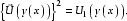Then the condition for the existence of an extremum should be defined on the basis of :It is not difficult to see that the extremum of (A1) is the sum of the extrema of its two squared components.

The theory of the calculus of variations makes clear that the extremum of a nonstandard function of the type F(y(x)) = F 1(y(x))F 2 (y(x)) is to be found on the basis of the linear combination:

G(y(x)) = F 1 (y(x)) + λ0 F 2 (y(x)),     (A2)

where λ0 is to be obtained from the ratio: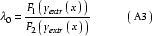and y extr (x) is defined using the conditions for extremum.

The functionals in (A1):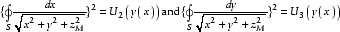can be represented as a product of two equal functions. Then, in the two cases of U 2 (y(x)) and U 3 (y(x)) a trivial value for λ0; λ0 = 1 renders the problem for extremum of the sum of the squares of the functions to an extremum of the sum of their power of one and in a sense – to the sum of the extrema of their moduluses.

The integrals in (A1) should be transformed in parametric form with arguments t:

x = x 1 (t); y = x 2 (t)

Introducing also the condition for a constant perimeter, the calculus of variations problem is presented in a near standard form – the extremum of :is to be found, having in view the limitation: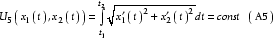The necessary conditions for the existence of an extremum are defined using the Lagrange function: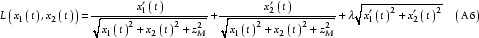where the Lagrange coefficient λ in this case is a constant, to be found according to the necessary conditions for extremum and the limitation (A5).

The necessary conditions for extremum can be defined by the Euler system of equations: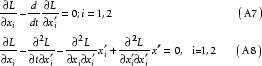with boundary conditions: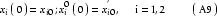It is known that the equations in this system are in linear relation. In this case, a calibration equation is recommended in the following form:For this specific problem, the limitation condition (A5) can be taken as calibration equation.

Lagrange function (A6) leads to a system of differential equations in parametric form: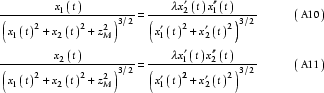with boundary conditions (A9).

### B. Sufficiency of the necessary conditions

Defining the conditions for sufficiency of the necessary conditions is a rather complicated procedure. Here, some general considerations can be noted.

1. 1.

The obtained extremum function is a solution of the Euler equation system – in this case equations (10) and (11).

2. 2.

If λ is considered a parameter of the extremum function family, for the accepted boundary conditions and varying λ, a central field of extremum functions is obtained. The numerical analysis can show that they do not cross, and that a conjugated point does not exist, therefore the Jacoby condition for sufficiency is met.

For the second partial derivatives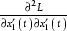and, taking into account Eq (9), we obtain: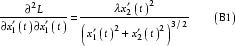and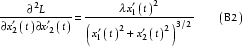It is obvious that (B-1) and (B-2) can have positive and negative values, which allows the conditions of Legendre to be met:;< 0

• a condition for maximum, specifically a weak maximum, which is achievable with curves having zero order proximity (proximity to ordinates):;> 0 - a condition for minimum

1. 4.

It can be verified, based on Eq. (9), that the third partial derivative: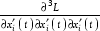exists, which is also a part of the sufficiency conditions.

### C. Field induced by a system of excitation coils

A relatively arbitrarily shaped contour L is considered (Fig. 1) with a current i(t) that excites an induced electrical field(x, y, z). The field is obtained from Eq. (4), or: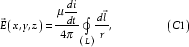whereis a vector linear element of the integration contour (L) and r is the distance from the elementto the point where(x, y, z) is computed.

Difficulties also appear for cases of contours in a relatively general position with respect to the coordinate axes . Such difficulties are not insurmountable, but should be dealt with depending on the specific problem in view.

The present investigation relates to fields induced by flat contours approximated by n linear segments (Fig. 3) located in the XOY and YOZ planes. Applying the calculus of variation approach, such an approximation is justified. The numerical solution (Fig. 2) suggests that any other type of approximation would involve computational difficulties without major impact on the practical results. The solution accuracy depends, of course, on the value of n. For the triangular and square shapes, n is defined and does not affect the accuracy.

For a system of k contours, the resulting induced field is obtained by superposition, based on the linear relation between(x, y, z) and di / dt, as evident from Eq. C1. In this case, three coils (L 1),(L 2) and (L 3) are involved, where (L 1) and (L 2) are symmetrically located in the XOY plane, and (L 3) is in the YOZ plane, symmetrically oriented with respect to the negative Z-axis. The region to be stimulated is located in the positive half-space defined by XOY and the positive Z-axis.(x, y, z) is to be obtained by solving the contour integrals along (L 1), (L 2) and (L 3):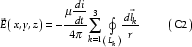After decomposition ofover the respective axes, the following relation is obtained:For the contours approximated by n segments (Fig. 3), sums of integrals are obtained for (L 1) and (L 2) respectively: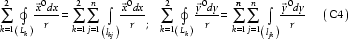and for (L 3):The segments l j are introduced in the contour integrals by the corresponding linear relations y = m jk x + n jk , k = 1, 2 ; j = 1,2,...,n in the XOY plane and z = m j y + n j in the YOZ plane.

The segments l j are introduced in the contour integrals by the corresponding linear relations y = m jk x + n jk , k = 1, 2 ; j = 1,2,...,n in the XOY plane and z = m j y + n j in the YOZ plane.

## References

1. Polson MJR, Barker AT, Freeston IL: Stimulation of nerve trunks with time-varying magnetic fields. Med Biol Eng Comput 1982, 20: 243–244.

2. Mills RK, Murray NM, Hess CW: Magnetic and electrical transcranial brain stimulation: physiological mechanisms and clinical applications. Neurosurg 1987, 20: 164–168.

3. Stuchly MA: Applications of time-varying magnetic fields in medicine. Crit Rev Biomed Eng 1990, 18: 89–124.

4. Ruohonen J, Virtanen J, Ilmoniemi RJ: Coil optimization for magnetic brain stimulation. Ann Biomed Eng 1997, 25: 840–849.

5. Mouchawar G, Bourland JD, Voorhees WD, Geddes LA: Stimulation of inspiratory motor nerves with a pulsed magnetic field (letter). Med Biol Eng Comput. 1990, 28: 613.

6. Geddes LA, Mouchawar G, Bourland JD, ElAbbady T, Nyenhius J: Magnetic (eddy-current) electroventilation in the dog. Ann Biomed Eng 1993, 21: 193–197.

7. Mouchawar G, Nyenhius J, Bourland JD, Geddes LA: Gidelines for energy-efficient coils: coils designed for magnetic stimulation of the heart. Electroenceph Clin Neurophysiol 1991,43(suppl):255–267.

8. Mouchawar G, Bourland JD, Nyenhius J, Geddes LA, Foster KS, Jones JT, Graber GP: Closed-chest cardiac stimulation with a pulsed magnetic field. Med Biol Eng Comput 1992, 30: 162–168.

9. Yamagushi M, Andoh T, Goto T, Hosono A, Kawakami T, Okumura F, Takenaka T, Yamamoto I: Effects of strong pulsed magnetic fields on the cardiac activity of an open chest dog. IEEE Trans Biomed Eng 1994, 41: 1188–1191. 10.1109/10.335868

10. Reilly JP: Magnetic field excitation of peripheral nerves and the heart: a comparison of thresholds. Med Biol Eng Comput 1991, 29: 571–579.

11. Cohen LG, Roth BJ, Nilsson J, Dang N, Panizza M, Bandinelli S, Friauf W, Hallet M: Effects of coil design on delivery of focal magnetic stimulation. Technical considerations. Electroenceph Clin Neurophysiol 1990, 75: 255–267. 10.1016/0013-4694(90)90105-S

12. Roth BJ, Maccabee PJ, Eberle LP, Amassian VE, Hallett M, Cadwell J, Anselmi GD, Tatarian GT: In vitro evaluation of a 4-leaf coil design for magnetic stimulation of peripheral nerve. Electroenceph Clin Neurophysiol 1994, 93: 255–267. 10.1016/0168-5597(94)90027-2

13. Ren C, Tarjan PP, Popovic DB: A novel electric design for electromagnetic stimulation – the slinky coil. IEEE Trans Biomed Eng 1995, 42: 918–925. 10.1109/10.412658

14. Zimmerman KP, Simpson RK: Slinky coils for neuromagnetic stimulation. Electroenceph Clin Neurophysiol 1996, 101: 145–152. 10.1016/0924-980X(95)00227-C

15. W-H Lin V, Hsiao IN, Dhaka V: Magnetic coil design considerations for functional magnetic stimulation. IEEE Trans Biomed Eng 2000, 47: 600–610. 10.1109/10.841332

16. Elsgolc LE: Calculus of variations. Pergamon Press, Oxford 1962.

17. Bliss GA: Lectures on the Calculus of Variations. The University of Chicago Press, Chicago, London 1963.

18. Akhiezer NI: The Calculus of Variations. Harwood Academic Publishers, Chur, Switzerland 1988.

Authors

## Authors’ original submitted files for images

Below are the links to the authors’ original submitted files for images.

## Rights and permissions

Reprints and Permissions

Papazov, S.P., Daskalov, I.K. Effect of Contour Shape of Nervous System Electromagnetic Stimulation Coils on the Induced Electrical Field Distribution. BioMed Eng OnLine 1, 1 (2002). https://doi.org/10.1186/1475-925X-1-1

• Accepted:

• Published:

• DOI: https://doi.org/10.1186/1475-925X-1-1

### Keywords

• Electrical Field Distribution
• Extremum Function
• Circular Coil
• Coil Shape
• Optimal Contour